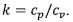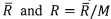milk26364325>試卷(2021/09/15)

# 110 年 - 110 國立臺灣大學_碩士班招生考試_工程科學及海洋工程學研究所甲組:熱工學#101265

【非選題】
1.

273.15 K = 0℃. Molecular weights of air and water are 28.97 kg/kmol and 18 kg/kmol, respectively. Universal gas constant = 8.314 kJ/kmol-K. I ton =1000 kg.
1. A regenerative power cycle with one open feedwater heater is given in Figure 1 where the operation conditions are summarized in Table 1. For a net power of 248 MW, determine (a) the temperature T2 in℃, (b) the quality x3, (c) the temperature T4, in ℃, (d) the temperature T5, in 'C. in℃, (e) the temperature T6 in ℃, (f) the temperature T7in℃, (g) the fraction of the total flow not entering the second turbine y, (h) thermal efficiency η, and (i) the mass flow rate of water m, in ton/hr. (36%)【非選題】
2.

2. A closed and rigid vessel containing air-water vapor mixture undergoes a cooling process with the volume of 2.172 m3, Fig.2. The operation conditions are summarized in Table 2. The dew point temperature of the initial state is 99.63℃. Air behaviors as an ideal gas with specific heat ratio of 1.4. Determine (a) the initial temperature of mixture Ti, in℃, (b) the initial pressure of mixture p1, in bar, (c) the mass of vapor, in kg, (d) the mass of dry air, in kg, (e) the temperature of mixture T2 when water vapor starts to condensate, in ℃,
(D) the pressure of the mixture P2, in bar, as the mixture temperature reaches T2, (g) the heat transfer during the cooling process, in kJ, (h) the entropy production during the cooling process, in kJ/K, if the vessel boundary always keeps at a constant temperature of T2. (32%)【非選題】
3.

3. For a closed simple compressible system operating with air as an ideal gas with molecular weight M as well as constant specific heats cp and cv where. The temperature T, pressure p, specific volume v and specific entropy s of two equilibrium states of this system are given as (T1, p1, v1, S1) and (T2, p2, v2, S2), respectively. The universal gas constant is. Please derive the following relations. (32%)

【題組】 (a) Tds = du + pdo and Tds = dh -vdp

【非選題】
4.【題組】(b)s2 -S1 =cvIn(T2/T1) +R(v2/v1) and s2 -s1=cp(T2/T1)-R(p2/p1)

【非選題】
5.【題組】

(c) For an isentropic process,【非選題】
6.【題組】

(d) The thermal eficiencyof a cold air-standard Otto cycle with the compression ratio r where its processes are modeled by a closed simple compressible system.

### 懸賞詳解

#### 國三地理下第一次

17. 企業在選擇生產基地的過程中，受到交通發展的影響，可 以將生產基地分散於不同國家，形成何種類型的生產方 式？ (A)時空收斂 (B)國際分工 (C)區...